# Dodecagram

Regular dodecagram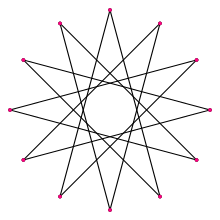A regular dodecagram
Type Regular star polygon
Edges and vertices 12
Schläfli symbol {12/5}
t{6/5}
Coxeter diagram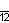Symmetry group Dihedral (D12)
Internal angle (degrees) 30°
Dual polygon self
Properties star, cyclic, equilateral, isogonal, isotoxal

A dodecagram is a star polygon that has 12 vertices. There is one regular form: {12/5}. A regular dodecagram has the same vertex arrangement as a regular dodecagon, which may be regarded as {12/1}.

The name dodecagram combine a numeral prefix, dodeca-, with the Greek suffix -gram. The -gram suffix derives from γραμμῆς (grammēs) meaning a line.

## Isogonal variations

A regular dodecagram can be seen as a quasitruncated hexagon, t{6/5}={12/5}. Other isogonal (vertex-transitive) variations with equal spaced vertices can be constructed with two edge lengths.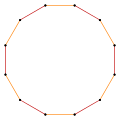t{6}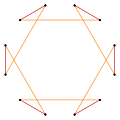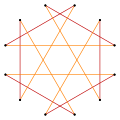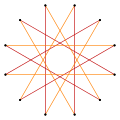t{6/5}={12/5}

## Dodecagrams as compounds

There are four regular dodecagram star figures, {12/2}=2{6}, {12/3}=3{4}, {12/4}=4{3}, and {12/6}=6{2}. The first is a compound of two hexagons, the second is a compound of three squares, the third is a compound of four triangles, and the fourth is a compound of six straight-sided digons.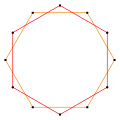2{6}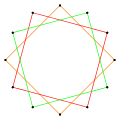3{4}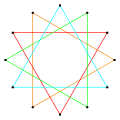4{3}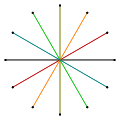6{2}

## Complete graph

Superimposing all the dodecagons and dodecagrams on each other – including the degenerate compound of six digons (line segments), {12/6} – produces the complete graph K12.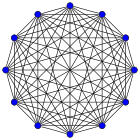## Regular dodecagrams in polyhedra

Dodecagrams can also be incorporated into uniform polyhedra. Below are the three prismatic uniform polyhedra containing regular dodecagrams.